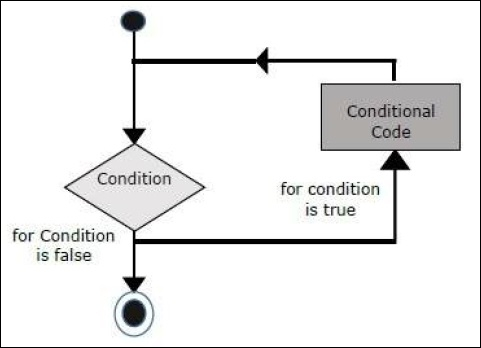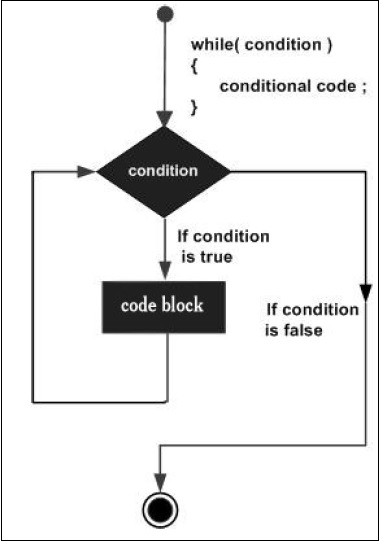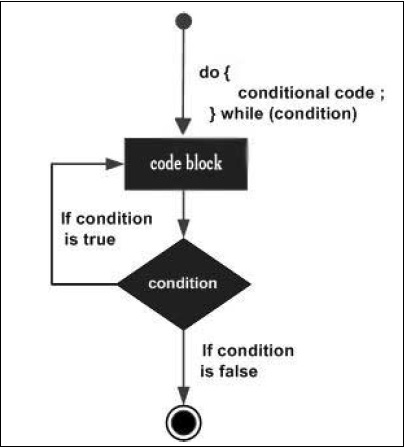# Looping through an array in Javascript

JavascriptFront End TechnologyWeb Development

There are a lot of ways to loop through an array in Javascript.

## For Loops in Javascript

Let’s start with them for a loop. There are 2 variations of the for loop in js. The first form is the init, condition, expr loop. This initializes the first statement, then on each iteration executes the expr and checks the condition.For example,

var step;
for (step = 0; step < 5; step++) {
console.log('Taking step ' + step);
}

This will give the output:

Taking step 0
Taking step 1
Taking step 2
Taking step 3
Taking step 4

There is another form of the for loop, the for in loop. The for...in statement iterates a specified variable over all the enumerable properties of an object. For each distinct property, JavaScript executes the specified statements. For example,

let person = {
name: "John",
age: 35
};

for (let prop in person) {
console.log(prop, a[prop]);
}

This will give the output:

name John
age 35

## The while loop in Javascript

The purpose of a while loop is to execute a statement or code block repeatedly as long as an expression is true. Once the expression becomes false, the loop terminates.For example,

let i = 0;
while (i < 5) {
console.log("Hello");
i = i + 1;
}

This will give the output:

Hello
Hello
Hello
Hello
Hello

## The do…while loop

The do...while loop is similar to the while loop except that the condition check happens at the end of the loop. This means that the loop will always be executed at least once, even if the condition is false.For example,

let i = 0;
do {
console.log("Hello");
i = i + 1;
} while (i < 5);

This will give the output −

Hello
Hello
Hello
Hello
Hello